# Week 3 - lecture 2

## TOP TOPIC

Date: 01/09/22

3.4. Birth and death chains
3.5. A markov chain from linear algebra
3.6. Counting and diameter lower bounds
3.7. Bottleneck Ratio

## Homework

1.* Letbe simple random walk on the integers, with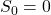. Show thatfor all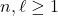. Hint: Prove the inequality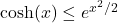for all real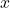, and use it to bound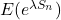.

2.*  Letbe a b-ary tree of height, that has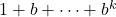vertices.  What are the best upper and lower bounds you can prove for the mixing time of lazy SRW on?

3. Let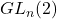be the set of invertible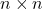matrices mod 2. Find the cardinality of. Hint: consider choosing the rows of the matrix one by one.

4.* Letbe a subset of the group of permutations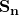. consider the Markov chain onobtained by picking a permutation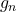inuniformly at random (independently of previous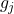), and defining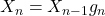.

(a)  Write a lower bound for the mixing time of this chain in terms ofand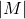.

(b) Suppose thatconsists of transpositions. What is the  smallest possible size offor which this chain is irreducible?

(c) Ifconsists of transpositions, can this chain be aperiodic?

(d) Suppose thatconsists of all transpositions, and we consider the lazy version of the chain above. Can you improve the lower bound from part (a) in this case?

## Reference

 Glasner, Shmuel.
Almost periodic sets and measures on the torus.
Israel J. Math. 32 (1979), no. 2-3, 161–-172.

 Berend, Daniel and Peres, Yuval.
Asymptotically dense dilations of sets on the circle.
J. London Math. Soc. (2) 47 (1993), no. 1, 1–-17.

 N. Alon and Y. Peres, "Uniform dilations'', Geometric and Functional Analysis, vol. 2, No. 1 (1992), 1–28.

 Nair, R. and Velani, S. L.
Glasner sets and polynomials in primes.
Proc. Amer. Math. Soc. 126 (1998), no. 10, 2835–-2840.